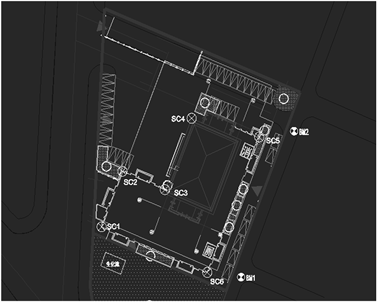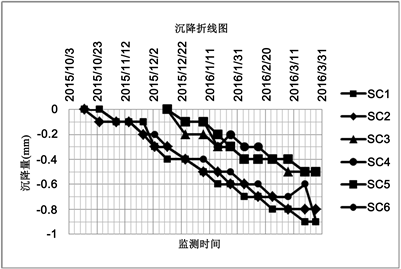﻿ 基于AR模型的建筑物沉降变形分析预测

基于AR模型的建筑物沉降变形分析预测Analysis and Prediction of Building Settlement Deformation Based on AR Model

Abstract: With the acceleration of China’s urbanization process, the rapid completion of high-rise buildings at the same time, its security issues are increasingly prominent. Autoregressive model (AR), as an important time series analysis method, can better reflect the rule of building settlement defor-mation change with time. In this paper, the settlement deformation of building is analyzed with an engineering example, and the autoregressive model is used to predict the amount of deformation.

1. 引言

2. 沉降观测方案设计

2.1. 水准测量方法

1) 精密水准测量

2) 精密高程三角测量

3) 液体静力水准测量

2.2. 监测精度及频率设计

2.3. 水准基点和沉降监测点的布设

1) 在建筑物主要墙角处或沿外墙每10 m~15 m处或每隔2~3根柱基上；

2) 沉降缝、伸缩缝、新旧建筑物接壤处的两侧；

3) 当建筑物出现裂缝时，布设在裂缝的两侧；

4) 建筑物沉降监测点标志的埋设位置应避开雨水管、窗台先、暖气管线和暖气片、电器开关等有碍设标语监测的障碍物，并应视立尺需要离开墙(柱)面和地面一定距离。所有监测点均应统一编号，并注记在各建筑物的平面图上。

3. 自回归模型

3.1. 自回归模型AR(p)

${X}_{t}={\phi }_{1}{X}_{t-1}+{\phi }_{2}{X}_{t-2}+\cdots +{\phi }_{p}{X}_{t-p}+{\epsilon }_{t}$ (1)

3.2. 参数估计

${V}_{t}={\phi }_{1}{X}_{t-1}+{\phi }_{2}{X}_{t-2}+\cdots +{\phi }_{p}{X}_{t-p}+{\epsilon }_{t}-{X}_{t}\left(t=p+1,p+2,\cdots ,p+n\right)$ (2)

$V=B\Phi -L$ (3)

$B=\left[\begin{array}{llll}{X}_{p}\hfill & {X}_{p-1}\hfill & \cdots \hfill & {X}_{1}\hfill \\ {X}_{p+1}\hfill & {X}_{p}\hfill & \cdots \hfill & {X}_{2}\hfill \\ ⋮\hfill & ⋮\hfill & \ddots \hfill & ⋮\hfill \\ {X}_{p+n-1}\hfill & {X}_{p+n-2}\hfill & \cdots \hfill & {X}_{N}\hfill \end{array}\right]$

$\Phi ={\left[\begin{array}{cc}\begin{array}{cc}{\phi }_{1}& {\phi }_{2}\end{array}& \begin{array}{cc}\cdots & {\phi }_{p}\end{array}\end{array}\right]}^{T}$

$L={\left[\begin{array}{cc}\begin{array}{cc}{X}_{p+1}& {X}_{p+2}\end{array}& \begin{array}{cc}\cdots & {X}_{p+n}\end{array}\end{array}\right]}^{T}$

${V}^{T}V=\mathrm{min}$ 下，模型参数最小二乘解为

$\stackrel{^}{\Phi }={\left({B}^{T}B\right)}^{-1}{B}^{T}L$ (4)

3.3. 模型阶数p的确定

${\phi }_{p}=0$ (5)

${\Omega }_{p-1}={\Omega }_{p}+R$ (6)

$F=\frac{R}{{\Omega }_{p}/\left(n-2p\right)}$ (7)

4. 工程实例Figure 1. Plane layout of settlement monitoring pointsTable 1. Accumulated settlement displacement monitoring results of monitoring points (unit: mm)Figure 2. Monitoring point settlement broken line diagramTable 2. Observation elevation records of each point in the 17th period (unit: m)Table 3. Comparison of predicted value and measured value (unit: m)

5. 结论

 孙雅荣, 吕品. 浅谈高层建筑的沉降观测[J]. 测绘与空间地理信息, 2006, 29(6): 104-106.

 缪海波, 殷坤龙, 柴波, 等. 基于非平稳时间序列分析的滑坡变形预测[J]. 地质科技, 2009, 28(4): 107-112.

 陈绍桔. 边坡非线性位移预测的动力系统自记忆模型[J]. 福建建筑, 2008(6): 154-157.

 兰孝奇. 时序分析在变形监测数据处理中的应用[J]. 工程勘察, 2004, 3(增刊): 61-62, 69.

 郑志勇, 张光华. 高大型建筑物沉降监测的方法及精度分析[J]. 地矿测绘, 2005, 21(3): 20-21.

 独知行, 靳奉祥, 冯遵德. 高层建筑物整体变形观测及分析方案[J]. 工程勘察, 2000(2): 55-58.

 赵言, 花向红, 翟高鹏. GM(1,1)与自回归模型在位移监测中的应用[J]. 城市勘测, 2011(3): 115-118.

 梅红, 岳东. 时间序列分析在变形监测数据处理中的应用[J]. 现代测绘, 2005, 28(6): 14-16.

 史玉峰, 孙保琪. 时间序列分析及其在变形数据分析中的应用[J]. 金属矿山, 2004(8): 13-15.

 陆立, 胡晓丽, 王春华. 用时间序列分析法进行建筑物沉降观测数据处理的研究[J]. 测绘科学, 2004, 29(6): 77-79.

Top